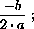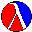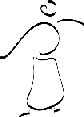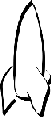# Section 10More on Processing Lists

The functions in section 9 consume lists that contain atomic data, especially numbers, symbols, and booleans. But functions must also be able to produce such lists. Furthermore, they must be able to consume and produce lists that contain structures. We discuss these cases in this section, and we continue practicing the use of the design recipe.

## 10.1  Functions that Produce Lists

Recall the function `wage` from section 2.3:

```;; `wage : number  ->  number`
;; to compute the total wage (at \$12 per hour)
;; of someone who worked for `h` hours
(define (wage h)
(* 12 h))
```

The `wage` function consumes the number of hours some employee worked and produces the weekly wage payment. For simplicity, we assume that all employees earn the same hourly rate, namely, \$12. A company, however, isn't interested in a function like `wage`, which computes the wage of a single employee. Instead, it wants a function that computes the wages for all of its employees, especially if there are a lot of them.

Call this new function `hours->wages`. It consumes a list that represents how many hours the employees of the company worked and must produce a list of the weekly wages they earned. We can represent both the input and the output as Scheme lists of numbers. Since we already have a data definition for the inputs and outputs, we can immediately start our function development:

```;; `hours->wages : list-of-numbers  ->  list-of-numbers`
;; to create a list of weekly wages from a list of weekly hours (`alon`)
(define (hours->wages alon) ...)
```

Next we need some examples of inputs and the corresponding outputs:

```empty
(cons 28 empty)
(cons 40 (cons 28 empty))
```

```empty
(cons 336 empty)
(cons 480 (cons 336 empty))
```
The outputs are obtained by calculating the wage for each item on the list to the left.

Given that `hours->wages` consumes the same class of data as, say, the function `sum`, and given that the shape of a function template depends only on the shape of the data definition, we can reuse the `list-of-numbers` template:

```(define (hours->wages alon)
(cond
[(empty? alon) ...]
[else ... (first alon) ... (hours->wages (rest alon)) ...]))
```

Starting with this template, we can turn to the most creative step of function development: the definition of the function body. Following our recipe, we consider each `cond`-line in isolation, starting with the simpler case. First, assume `(empty? alon)` is true, which means that the input is `empty`. The answer in this case is `empty`:

```(define (hours->wages alon)
(cond
[(empty? alon) empty]
[else ... (first alon) ... (hours->wages (rest alon)) ...]))
```

Second, assume that `alon` was `cons`tructed from a number and a list of numbers. The expressions in the second line remind us of this assumption, and the recipe tells us that we should state explicitly what they compute:

1. `(first alon)` yields the first number on `alon`, which is the first number of hours worked; and

2. `(hours->wages (rest alon))` reminds us that ```(rest alon)``` is a list and can be processed by the very function we are defining. According to the purpose statement, the expression computes the list of wages for the rest of the list of hours, and we may assume this relationship in our construction -- even though the function is not yet completely defined.

From here it is a short step to the complete function definition. Since we already have the list of wages for all but the first item of `alon`, the function must do two things to produce an output for the entire list of hours:

1. Compute the weekly wage for the first number of hours.

2. Construct a list that represents all weekly wages for `alon`, using the first weekly wage and the list of weekly wages for ```(rest alon)```.

For the first part, we reuse `wage`. For the second, we `cons` the two pieces of information together into one list:

```(cons (wage (first alon)) (hours->wages (rest alon)))
```

And with that, we have a complete function. It is shown in figure 27. To finish the design of the function, we must still test it.

 ```;; `hours->wages : list-of-numbers  ->  list-of-numbers` ;; to create a list of weekly wages from a list of weekly hours (`alon`) (define (hours->wages alon) (cond [(empty? alon) empty] [else (cons (wage (first alon)) (hours->wages (rest alon)))])) ;; `wage : number  ->  number` ;; to compute the total wage (at \$12 per hour) ;; of someone who worked for `h` hours (define (wage h) (* 12 h)) ``` Figure 27:  Computing weekly wages

Exercise 10.1.1.   How do we have to change the function in figure 27 if we want to give everyone a raise to \$14?Solution

Exercise 10.1.2.   No employee could possibly work more than 100 hours per week. To protect the company against fraud, the function should check that no item of the input list of `hours->wages` exceeds 100. If one of them does, the function should immediately signal the error `"too many hours"`.

How do we have to change the function in figure 27 if we want to perform this basic reality check?Solution

Exercise 10.1.3.

Develop `convertFC`. The function converts a list of Fahrenheit measurements to a list of Celsius measurements.Solution

Exercise 10.1.4.   Develop the function `convert-euro`, which converts a list of U.S. dollar amounts into a list of euro amounts. Assume the exchange rate is 1.22 euro for each dollar.

Generalize `convert-euro` to the function `convert-euro-1`, which consumes an exchange rate and a list of dollar amounts and converts the latter into a list of euro amounts.Solution

Exercise 10.1.5.   Develop the function `eliminate-exp` to eliminate expensive toys. The function consumes a number, called `ua`, and a list of toy prices, called `lotp`, and produces a list of all those prices in `lotp` that are below or equal to `ua`. For example,32

```(eliminate-exp 1.0 (cons 2.95 (cons .95 (cons 1.0 (cons 5 empty)))))
;; expected value:
(cons .95 (cons 1.0 empty))
```Solution

Exercise 10.1.6.   Develop the function `name-robot`, which consumes a list of toy descriptions (names) and produces an equivalent list of more accurate descriptions. Specifically, it replaces all occurrences of `'robot` with `'r2d2` and otherwise retains the toy descriptions in the same order.

Generalize `name-robot` to the function `substitute`. The new function consumes two symbols, called `new` and `old`, and a list of symbols. It produces a new list of symbols by substituting all occurrences of `old` by `new`. For example,

```(substitute 'Barbie 'doll (cons 'robot (cons 'doll (cons 'dress empty))))
;; expected value:
(cons 'robot (cons 'Barbie (cons 'dress empty)))
```Solution

Exercise 10.1.7.   Develop the function `recall` to eliminate specific toys from a list. The function consumes the name of a toy, called `ty`, and a list of names, called `lon`, and produces a list of names that contains all components of `lon` with the exception of `ty`. For example,

```(recall 'robot (cons 'robot (cons 'doll (cons 'dress empty))))
;; expected value:
(cons 'doll (cons 'dress empty))
```Solution

Exercise 10.1.8.   Develop `quadratic-roots`, which solves quadratic equations: see exercises 4.4.4 and 5.1.4. The function accepts the coefficients `a`, `b`, and `c`. The computations it performs depend on the input:

1. if a = 0, its output is `'degenerate`.

2. if b2 < 4 · a · c, the quadratic equation has no solution; `quadratic-roots` produces `'none` in this case.

3. if b2 = 4 · a · c, the equation has one solution:4. if b2 > 4 · a · c, the equation has two solutions:andthe result is a list of two numbers: the first solution followed by the second solution.

Test the function with the examples from exercises 4.4.4 and 5.1.4. First decide the answer for each example, then determine it with DrScheme.Solution

Exercise 10.1.9.   The cash registers at many grocery stores talk to customers. The register's computer receives the number of cents that the customer must pay and then builds a list with the following five items:

1. the dollar amount;

2. the symbol `'dollar` if the dollar amount is `1` and `'dollars` otherwise;

3. the symbol `'and`;

4. the cent amount; and

5. the symbol `'cent` if the cent amount is 1 and `'cents` otherwise.

Develop the function `controller`, which consumes a number and produces a list according to the above description. For example, if the amount is \$1.03, then the cash register evaluates `(controller 103)`:

```(controller 103)
;; expected value:
(cons 1 (cons 'dollar (cons 'and (cons 3 (cons 'cents empty)))))
```

Hint: Scheme provides the arithmetic operations `quotient` and `remainder`, which produce the quotient and remainder of the expression n/m for integers n and m, respectively.Sound Files

Once the controller returns the correct list for amounts whose dollar and cent amounts are between 0 and 20, test the controller with a computer that can speak. Set the teachpack to sound.ss, which makes two operations available: `speak-word` and `speak-list`. The first accepts a symbol or a number, the second a list of symbols and numbers. Both pronounce the symbols they consume. Evaluate the following expressions `(speak-word 1)`, ```(speak-list (cons 1 (cons 'dollar empty)))```, and ```(speak-list (cons 'beautiful (cons 'lady empty)))``` to understand how the operations operate.

Simple Challenge: The sound teachpack contains only the sounds for the numbers `0` through `20` and `30`, `40`, `50`, `60`, `70`, `80`, and `90`. Because of this restriction, this challenge problem works only on amounts with cents and dollars between `0` to `20`. Implement a controller that deals with arbitrary amounts between 0 and 99.99.Solution

## 10.2  Lists that Contain Structures

The representation of an inventory as a list of symbols or a list of prices is naive. A sales clerk in a toy store needs to know not only the name of the toy, but also its price, and possibly other attributes like warehouse availability, delivery time, or even a picture of the item. Similarly, representing the personnel's work week as a list of hours is a bad choice. Even the printing of a paycheck requires more information about the employee than the hours worked per week.

Fortunately, the items of lists do not have to be atomic values. Lists may contain whatever values we want, especially structures. Let's try to make our toy store inventory functions more realistic. We start with the structure and the data definition of a class of inventory records:

```(define-struct ir (name price))
```

An inventory-record (short: ir) is a structure:

`(make-ir s n)`

where `s` is a symbol and `n` is a (positive) number.

Most important, we can define a class of lists that represent inventories much more realistically:

An inventory is either

1. `empty` or

2. `(cons ir inv)`
where `ir` is an inventory record and `inv` is an inventory.

While the shape of the list definition is the same as before, its components are defined in a separate data definition. Since this is our first such data definition, we should make up some examples before we proceed.

The simplest example of an inventory is `empty`. To create a larger inventory, we must create an inventory record and `cons` it onto another inventory:

```(cons (make-ir 'doll 17.95)
empty)
```

From here, we can create yet a larger inventory listing:

```(cons (make-ir 'robot 22.05)
(cons (make-ir 'doll 17.95)
empty))
```

Now we can adapt our inventory-processing functions. First look at `sum`, the function that consumes an inventory and produces its total value. Here is a restatement of the basic information about the function:

```;; `sum : inventory  ->  number`
;; to compute the sum of prices on `an-inv`
(define (sum an-inv) ...)
```

For our three sample inventories, the function should produce the following results: `0`, `17.95`, and `40.0`.

Since the data definition of inventories is basically that of lists, we can again start from the template for list-processing functions:

```(define (sum an-inv)
(cond
[(empty? an-inv) ...]
[else ... (first an-inv) ... (sum (rest an-inv)) ...]))
```

Following our recipe, the template only reflects the data definition of the input, not that of its constituents. Therefore the template for `sum` here is indistinguishable from that in section 9.5.

For the definition of the function body, we consider each `cond`-line in isolation. First, if `(empty? an-inv)` is true, `sum` is supposed to produce `0`. Hence the answer expression in the first `cond`-line is obviously `0`.

 ```(define (sum an-inv) (cond [(empty? an-inv) 0] [else (+ (ir-price (first an-inv)) (sum (rest an-inv)))])) ``` Figure 28:  Computing the value of an inventory

Second, if `(empty? an-inv)` is false, in other words, if `sum` is applied to a `cons`tructed inventory, the recipe requires us to understand the purpose of two expressions:

1. `(first an-inv)`, which extracts the first item of the list; and

2. `(sum (rest an-inv))`, which extracts the rest of `an-inv` and then computes its cost with `sum`.

To compute the total cost of the entire input `an-inv` in the second case, we must determine the cost of the first item. The cost of the first item may be obtained via the selector `ir-price`, which extracts the price from an inventory record. Now we just add the cost of the first item and the cost of the rest of the inventory:

```(+ (ir-price (first an-inv))
(sum (rest an-inv)))
```

The complete function definition is contained in figure 28.

Exercise 10.2.1.   Adapt the function `contains-doll?` so that it consumes inventories instead of lists of symbols:

```;; `contains-doll? : inventory  ->  boolean`
;; to determine whether `an-inv` contains a record for `'doll`
(define (contains-doll? an-inv) ...)
```

Also adapt the function `contains?`, which consumes a symbol and an inventory and determines whether an inventory record with this symbol occurs in the inventory:

```;; `contains? : symbol inventory  ->  boolean`
;; to determine whether `inventory` contains a record for `asymbol`
(define (contains? asymbol an-inv) ...)
```Solution

 Item Price Image robot 29.95robot 29.95robot 29.95Figure 29:  A table of toys

Exercise 10.2.2.   Provide a data definition and a structure definition for an inventory that includes pictures with each object. Show how to represent the inventory listing in figure 29.33

Develop the function `show-picture`. The function consumes a symbol, the name of a toy, and one of the new inventories. It produces the picture of the named toy or `false` if the desired item is not in the inventory. Pictures of toys are available on the Web.Solution

Exercise 10.2.3.   Develop the function `price-of`, which consumes the name of a toy and an inventory and produces the toy's price.Solution

Exercise 10.2.4.   A phone directory combines names with phone numbers. Develop a data definition for phone records and directories. Using this data definition develop the functions

1. `whose-number`, which determines the name that goes with some given phone number and phone directory, and

2. `phone-number`, which determines the phone number that goes with some given name and phone directory.Solution

Suppose a business wishes to separate all those items that sell for a dollar or less from all others. The goal might be to sell these items in a separate department of the store. To perform this split, the business also needs a function that can extract these items from its inventory listing, that is, a function that produces a list of structures.

Let us name the function `extract1` because it creates an inventory from all those inventory records whose price item is less than or equal to `1.00`. The function consumes an inventory and produces one with items of appropriate prices. Thus the contract for `extract1` is easy to formulate:

```;; `extract1 : inventory  ->  inventory`
;; to create an `inventory` from `an-inv` for all
;; those items that cost less than \$1
(define (extract1 an-inv) ...)
```

We can reuse our old inventory examples to make examples of `extract1`'s input-output relationship. Unfortunately, for these three examples it must produce the empty inventory, because all prices are above one dollar. For a more interesting input-output example, we need an inventory with more variety:

```(cons (make-ir 'dagger .95)
(cons (make-ir 'Barbie 17.95)
(cons (make-ir 'key-chain .55)
(cons (make-ir 'robot 22.05)
empty))))
```

Out of the four items in this new inventory, two have prices below one dollar. If given to `extract1`, we should get the result

```(cons (make-ir 'dagger .95)
(cons (make-ir 'key-chain .55)
empty))
```

The new listing enumerates the items in the same order as the original, but contains only those items whose prices match our condition.

The contract also implies that the template for `extract1` is identical to that of `sum`, except for a name change:

```(define (extract1 an-inv)
(cond
[(empty? an-inv) ...]
[else ... (first an-inv) ... (extract1 (rest an-inv)) ...]))
```

As always, the difference in outputs between `sum` and `extract1` does not affect the template derivation.

 ```;; `extract1 : inventory  ->  inventory` ;; to create an `inventory` from `an-inv` for all ;; those items that cost less than \$1 (define (extract1 an-inv) (cond [(empty? an-inv) empty] [else (cond [(<= (ir-price (first an-inv)) 1.00) (cons (first an-inv) (extract1 (rest an-inv)))] [else (extract1 (rest an-inv))])])) ``` Figure 30:  Extracting dollar items from an inventory

For the definition of the function body, we again analyze each case separately. First, if `(empty? an-inv)` is true, then the answer is clearly `empty`, because no item in an empty store costs less than one dollar. Second, if the inventory is not empty, we first determine what the expressions in the matching `cond`-clause compute. Since `extract1` is the first recursive function to produce a list of structures, let us look at our interesting example:

```(cons (make-ir 'dagger .95)
(cons (make-ir 'Barbie 17.95)
(cons (make-ir 'key-chain .55)
(cons (make-ir 'robot 22.05)
empty))))
```

If `an-inv` stands for this inventory,

```(first an-inv) = (make-ir 'dagger .95)

(rest an-inv) = (cons (make-ir 'Barbie 17.95)
(cons (make-ir 'key-chain .55)
(cons (make-ir 'robot 22.05)
empty)))
```

Assuming `extract1` works correctly, we also know that

```(extract1 (rest an-inv)) = (cons (make-ir 'key-chain .55)
empty)
```

In other words, the recursive application of `extract1` produces the appropriate selection from the rest of `an-inv`, which is a list with a single inventory record.

To produce an appropriate inventory for all of `an-inv`, we must decide what to do with the first item. Its price may be more or less than one dollar, which suggests the following template for the second answer:

```... (cond
[(<= (ir-price (first an-inv)) 1.00) ...]
[else ...]) ...
```

If the first item's price is one dollar or less, it must be included in the final output and, according to our example, should be the first item on the output. Translated into Scheme, the output should be a list whose first item is `(first an-inv)` and the rest of which is whatever the recursion produces. If the price is more than one dollar, the item should not be included. That is, the result should be whatever the recursion produces for the `rest` of `an-inv` and nothing else. The complete definition is displayed in figure 30.

Exercise 10.2.5.   Define the function `extract>1`, which consumes an inventory and creates an inventory from those records whose prices are above one dollar.Solution

Exercise 10.2.6.   Develop a precise data definition for inventory1, which are inventory listings of one-dollar stores. Using the new data definition, the contract for `extract1` can be refined:

```;; `extract1 : inventory  ->  inventory1`
(define (extract1 an-inv) ...)
```

Does the refined contract affect the development of the function above?Solution

Exercise 10.2.7.   Develop the function `raise-prices`, which consumes an inventory and produces an inventory in which all prices are raised by 5%.Solution

Exercise 10.2.8.   Adapt the function `recall` from exercise 10.1.7 for the new data definition of inventory. The function consumes the name of a toy `ty` and an inventory and produces an inventory that contains all items of the input with the exception of those labeled `ty`.Solution

Exercise 10.2.9.   Adapt the function `name-robot` from exercise 10.1.6 for the new data definition of inventory. The function consumes an inventory and produces an inventory with more accurate names. Specifically, it replaces all occurrences of `'robot` with `'r2d3`.

Generalize `name-robot` to the function `substitute`. The new function consumes two symbols, called `new` and `old`, and an inventory. It produces a new inventory by substituting all occurrences of `old` with `new` and leaving all others alone.Solution

## 10.3  Extended Exercise: Moving Pictures

In sections 6.6 and 7.4, we studied how to move individual shapes. A picture, however, isn't just a single shape but a whole collection of them. Considering that we have to draw, translate, and clear pictures, and that we may wish to change a picture or manage several pictures at the same time, it is best to collect all of the parts of a picture into a single piece of data. Because pictures may consist of a varying number of items, a list representation for pictures naturally suggests itself.

Exercise 10.3.1.   Provide a data definition that describes the class of lists of `shape`s. The class of `shape`s was defined in exercise 7.4.1.

Create a sample list that represents the face of figure 10.3.6 and name it `FACE`. Its basic dimensions are gathered in the following table:

 shape position size(s) color circle (50,50) 40 red rectangle (30,20) 5 × 5 blue rectangle (65,20) 5 × 5 blue rectangle (40,75) 20 × 10 red rectangle (45,35) 10 × 30 blue
The table assumes a canvas of size 300 by 100.

Develop the template `fun-for-losh`, which outlines functions that consume a `list-of-shapes`.Solution

Exercise 10.3.2.   Use the template `fun-for-losh` to develop the function `draw-losh`. It consumes a `list-of-shapes`, draws each item on the list, and returns `true`. Remember to use `(start n m)` to create the canvas before the function is used.Solution

Exercise 10.3.3.   Use the template `fun-for-losh` to develop `translate-losh`. The function consumes a `list-of-shapes` and a number `delta`. The result is a list of shapes where each of them has been moved by `delta` pixels in the x direction. The function has no effect on the canvas.Solution

Exercise 10.3.4.   Use the template `fun-for-losh` to develop `clear-losh`. The function consumes a `list-of-shapes`, erases each item on the list from the canvas, and returns `true`.Solution

Exercise 10.3.5.   Develop the function `draw-and-clear-picture`. It consumes a `picture`. Its effect is to draw the picture, sleep for a while, and to clear the picture.Solution

Exercise 10.3.6.   Develop the function `move-picture`. It consumes a number (`delta`) and a `picture`. It draws the picture, sleeps for a while, clears the picture and then produces a translated version. The result should be moved by `delta` pixels.

Test the function with expressions like these:

```(start 500 100)

(draw-losh
(move-picture -5
(move-picture 23
(move-picture 10 FACE))))

(stop)
```

This moves `FACE` (see exercise 10.3.1) by `10`, `23`, and `-5` pixels in the x direction.Solution

When the function is fully tested, use the teachpack arrow.ss and evaluate the expression:

```(start 500 100)

(control-left-right FACE 100 move-picture draw-losh)
```

The last one creates a graphical user interface that permits users to move the shape `FACE` by clicking on arrows. The shape then moves in increments of `100` (right) and `-100` (left) pixels. The teachpack also provides arrow controls for other directions. Use them to develop other moving pictures.

32 Since we don't know yet how to compare two lists with a function, we use the old style of specifying examples and tests.

33 Thanks to Mr. John Clements for drawing these pictures.